# Spread Spectrum - 1.0 English

## Clocking Wizard for Versal ACAP LogiCORE IP Product Guide (PG321)

Document ID
PG321
Release Date
2022-04-20
Version
1.0 English

When Spread Spectrum (SS) is selected, CLK_OUT3 and CLK_OUT4 are not available. Divide values of these outputs are used for SS modulation frequency generation. There are four modes available for Spread Spectrum Mode:

• DOWN_LOW
• DOWN_HIGH
• CENTER_LOW
• CENTER_HIGH

The available modulation frequency range is 25 – 250 kHz.

Spread Spectrum calculation details are described in the following figures:

Figure 1. Spread Spectrum Mode (Center Spread)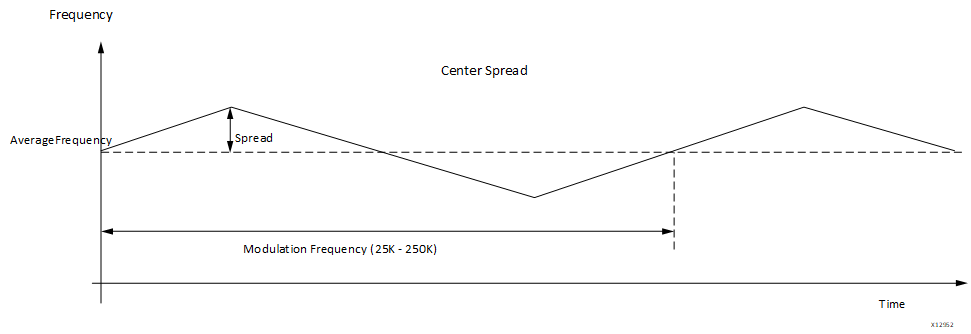Figure 2. Spread Spectrum Mode (Down Spread)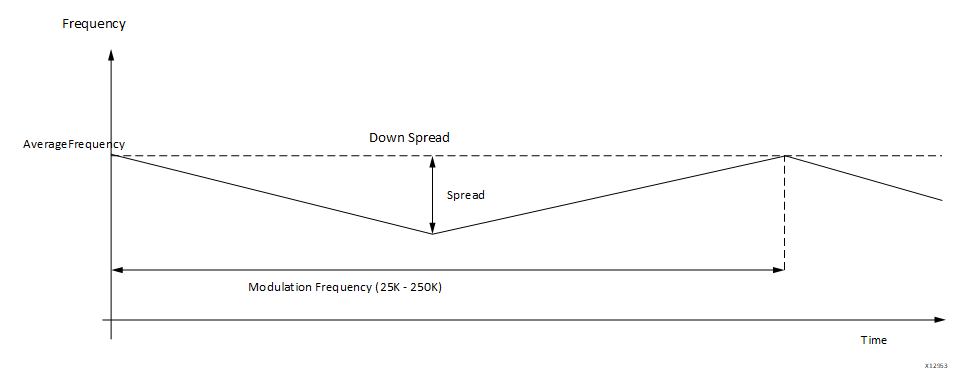Note: Input_clock_frequency is in Hz.

• If (SS_Mode = CENTER_HIGH):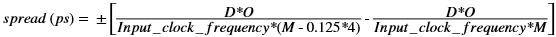• If (SS_Mode = CENTER_LOW):• If (SS_Mode = DOWN_HIGH):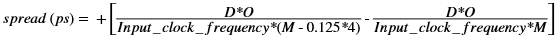• If (SS_Mode = DOWN_LOW):In the above equatons, M is CLKFBOUT_MULT_F, D is DIVCLK_DIVIDE, and O is respective CLKOUTX_DIVIDE.

For Modulation Frequency:
• O2 and O3 are calculated by the write_device_image in implementation. Same calculation is done in the wizard to get actual modulation frequency value.
• Then based on what O2 and O3 is calculated, the actual modulation frequency is calculated:
• If (SS_Mode = CENTER_HIGH or SS_Mode = CENTER_LOW)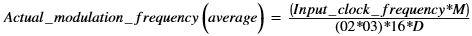• If (SS_Mode = DOWN_HIGH)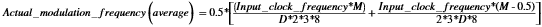• If (SS_Mode = DOWN_LOW)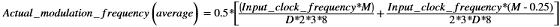Important: Actual modulation frequency may deviate within +/- 10% of the requested modulation frequency for some settings.